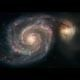# 42 as the sum of three cubes

In March, I wrote about the successful effort, after 64 years of computational striving, of finding integer solutions to the problem x3+y3+z3=33. This equation, along with x3+y3+z3=42, were the only two equations for which neither a solution nor a proof that no solution exists had been found for the right hand side being below 100. Finding that solution meant that only the 42 problem remained unsolved.

Now 42 problem too has been solved and in a nod to Douglas Adams, Andrew Sutherland (MIT) and Andrew Booker (Bristol), the finders of the solution, announced it on webpages titled Life, the Universe and Everything.

Every cube of a whole number is within one of a multiple of nine, which means that a sum of three cubes must be within three of a multiple of nine. So numbers of the form 9𝑘+49k+4 or 9𝑘+59k+5 cannot be written as the sum of three cubes.

In 1992, Roger Heath-Brown conjectured that every other whole number can be written as the sum of three cubes, in infinitely many different ways. Mathematicians on the whole seem to have been convinced by Heath-Brown’s argument that this ought to be true – but actually finding ways to write any particular number as a sum of three cubes remains a difficult problem.

The solution is:

x = −80,538,738,812,075,974
y = +80,435,758,145,817,515
z = +12,602,123,297,335,631

Now the only unsolved numbers below 1000 are 114, 165, 390, 579, 627, 633, 732, 906, 921, and 975.

The key question that was not answered is which of these three numbers provide the key to life, which provides the key to the universe, and which to everything else.

Here Brooker explains what was involved.

(Via Rob Beschizza.)

1.consciousness razor says

The key question that was not answered is which of these three numbers provide the key to life, which provides the key to the universe, and which to everything else.

“Life” is obviously negative and “everything” could not be less than the “universe,” so this must be it:
Life = (−80,538,738,812,075,974)^3
Universe = (12,602,123,297,335,631)^3
Everything = (80,435,758,145,817,515)^3
I’m glad we finally figured that out.

2.Mobius says

Dang. I was so close. If I just hadn’t failed to carry the 1 in one of my calculations.